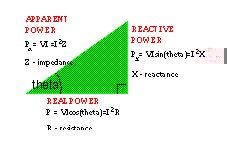## Electrical engineering concepts

Three phase system

At the large-scale, alternating-current (ac) electricity is generated and transmitted/distributed not as a single sinusoidal energy waveform, but as three. In fact any number of phase-displaced waveforms are possible, but in the technical/economic trade-off the three phase system is optimal.

A three phase system can be observed as any system of three voltages (or currents) in which the voltage (current) magnitudes and phase angles have a fixed relationship with each other. A balanced three phase system is one in which the magnitude of the three voltages is constant and the phase displacement is constant and equal to 120°.

Power factor

The power factor is dependent upon the characteristics of the electrical appliances connected and the relationship between the the amount of real and reactive power which is absorbed.In the power triangle diagram, cos(theta) is the power factor and it indicates the relative size of the reactive power component measured in Volt-Amperes-Reactive (VAR).

When the impedance of the circuit is purely resistive, there is no reactive component and the apparent power is equivalent to the real power (i.e. cos(theta)=0). When reactive components are introduced (inductance or capacitance) the impedance becomes a complex quantity with a resistive and a reactive component:

• impedance Z=R+jX

The reactive power component normally results from inductance present in the circuit. The inductor is a fundamental component of electrical networks and, in practice, inductance is unavoidable in standard equipment such as transformers, motors, and even cables. Inductance can be conceived as a circuit component which acts to oppose any change in the current flowing through it. The power triangle also reflects this phenomenon because the power factor angle, theta, represents the amount by which the current lags the voltage in an inductive circuit.

Therefore electrical circuits operate at a non-ideal (<1.0) power factor and one which is termed lagging (due to inductance). There is therefore a reactive power component which, whilst being the mathematically termed "imaginary" component, causes increased current flow and heating effects in wires and appliances (e.g. pump/fan motors).

The reduction of the reactive power component is desirable on technical and energy-efficiency grounds. Furthermore the electricity supply utility tariff structure penalises the reactive component of electrical load.